# OMAC: One-Key CBC MAC

OMAC is a blockcipher-based message authentication code designed and analyzed by me and Kaoru Kurosawa.

OMAC is a simple variant of the CBC MAC (Cipher Block Chaining Message Authentication Code). OMAC stands for One-Key CBC MAC.

OMAC allows and is secure for messages of any bit length (while the CBC MAC is only secure on messages of one fixed length, and the length must be a multiple of the block length). Also, the efficiency of OMAC is highly optimized. It is almost as efficient as the CBC MAC.

``NIST Special Publication 800-38B Recommendation for Block Cipher Modes of Operation: the CMAC Mode for Authentication'' has been finalized on May 18, 2005. This Recommendation specifies CMAC, which is equivalent to OMAC (OMAC1).

 OMAC: Brief description

OMAC is a generic name for OMAC1 and OMAC2. OMAC1 is described first. OMAC1 is equivalnent to CMAC.

 OMAC1:

Before using OMAC1, you have to choose a blockcipher E and a tag length t .

• E may be the AES, Camellia, Triple-DES, or whatever blockcipher you like. Suppose that the key length of E is k bits and the block length is n bits.
• The tag length t may be any integer between 1 and n , depending on your application (but make sure to retain adequate security).

Then you have to share a secret key with your partner. Let K be the k-bit OMAC1 key, which is just the key for E .

Pre-processing: The following steps can be done without the message.

1. First, encrypt n-bit 0 (denoted by 0 n ) to compute L . That is, let L be E (K, 0 n) .
2. Check if the most significant bit of L is 0 .
• If it is, let L.u be L<<1 , where L<<1 denotes a shift in which bits increase in significance with the most significant bit being lost and a zero coming into the least significant bit. See the following figure (Also available in [.ps] and [.pdf]).• Otherwise, let L.u be (L<<1) xor Constant , where Constant is the n-bit constant. If n=128 , then Constant is 0x00000000000000000000000000000087, and if n=64 , then Constant is 0x000000000000001b, where bits are presented as hexadecimal values with their most significant bits to the left.
3. Check if the most significant bit of L.u is 0 .
• If it is, let L.u 2 be (L.u)<<1 .
• Otherwise, let L.u 2 be ((L.u)<<1) xor Constant , where Constant is the same as above.
4. Save L.u and L.u 2 .

Tag-generation: Let M be the message. Break M into blocks M, M, ..., M[m] , where each M[i] (i = 1 ,..., m-1) is n bits. The last message block M[m] may have fewer than n bits (but it has 0 bits only if the message M is empty).

1. Let Y be 0 n .
2. For i = 1 to m-1 do : let Y[i] be E(K, M[i] xor Y[i-1]) .
3. Check if the bit length of the last message block M[m] is n bits.
• If it is, let X[m] be M[m] xor Y[m-1] xor L.u .
• Otherwise, let M[m] be M[m] 1 0 (n-1-(bit length of M[m])) . That is, append a 1 and then append the minimum number of 0s, so that the total length becomes n bits. Let X[m] be M[m] xor Y[m-1] xor L.u 2 .
4. Let T be E(K, X[m]) .
5. Let Tag be the t-bit truncation of T .
6. Return Tag.

Note that if the message length is a positive multiple of n , then L.u is used. Otherwise 10 i padding and L.u 2 are used. If the message is an empty string, then you have to append 10 n-1 and use L.u 2 .

Here is the algorithmical description in pseudocode (Also available in [.ps] and [.pdf]).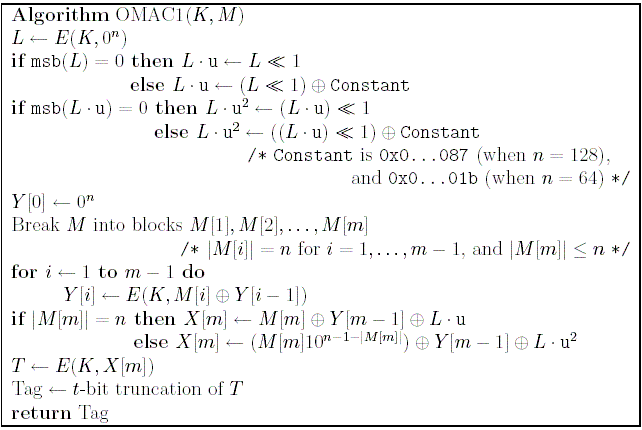Here are illustrations (Also available in [.ps] and [.pdf]).Tag-verification: Suppose that you have received a message-tag pair (M, Tag ') . To check if (M, Tag ') is authentic, first, compute the tag Tag for the message M using the above Tag-generation and your own secret key. If Tag ' = Tag then M is authentic. Otherwise, M is inauthentic.

 OMAC2:

Next, OMAC2 is described. In OMAC1, we use L.u and L.u 2 . In OMAC2, we use L.u and L.u -1 . To compute L.u -1 , first, check if the least significant bit of L is 0 .

• If it is, let L.u -1 be L>>1 , where L>>1 denotes a shift in which bits decreases with the least significant bit being lost and a zero coming into the most significant bit. See the following figure (Also available in [.ps] and [.pdf]).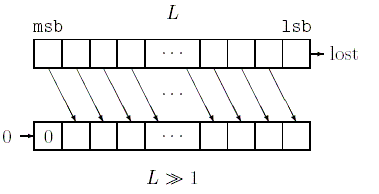• Otherwise, let L.u -1 be (L>>1) xor Constant ' , where Constant ' is the n-bit constant. If n=128 , then Constant ' is 0x80000000000000000000000000000043, and if n=64 , then Constant ' is 0x800000000000000d.

Here is the algorithmical description in pseudocode (Also available in [.ps] and [.pdf]).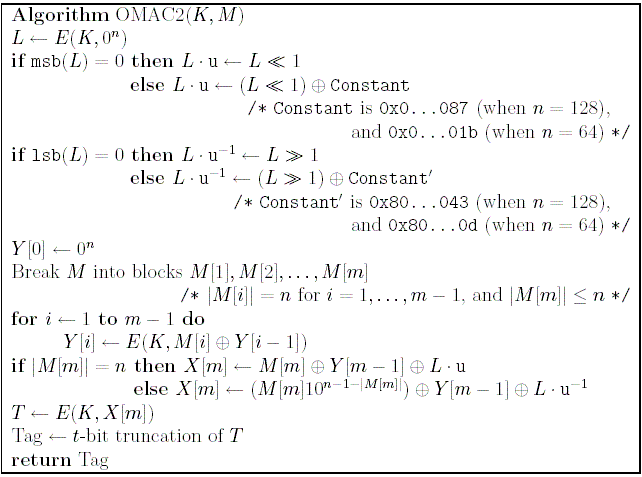OMAC: Documents
T. Iwata and K. Kurosawa. OMAC: One-Key CBC MAC. Fast Software Encryption, FSE 2003, LNCS 2887, pp. 129--153. Springer-Verlag (February 24, 2003, Lund, Sweden). Updated version is posted on IACR ePrint (March 10, 2003). Available in [.pdf].
• Documents submitted to NIST:
• T. Iwata and K. Kurosawa. OMAC: One-Key CBC MAC. NIST submission (December 20, 2002). Available in [.pdf].
• T. Iwata and K. Kurosawa. OMAC test vectors. NIST submission (December 20, 2002). Available in [.pdf].
• T. Iwata and K. Kurosawa. OMAC: One-Key CBC MAC --- addendum. NIST submission (March 10, 2003) Available in [.pdf].
• T. Iwata and K. Kurosawa. Stronger security bounds for OMAC, TMAC and XCBC. Comment to NIST (April 30, 2003). Available in [.pdf].
• T. Iwata. Comparison of CBC MAC variants and comments on NIST's consultation paper. Comment to NIST (May 5, 2003). Available in [.pdf].

 OMAC: Presentations
• OMAC: One-Key CBC MAC. Presented at FSE 2003 (February 25, 2003, Lund, Sweden). Available in [.pdf].
• Six conditions in OMAC-family are tight. Rump Session talk at FSE 2003 (February 24, 2003, Lund, Sweden). Available in [.pdf].
• A survey of CBC MAC variants. Presented at the workshop held in Tokyo (June 3, 2003, Tokyo, Japan). Available in [.pdf].

 OMAC: Test vectors

Test vectors are given for implementations with the AES as the underlying blockcipher (See also ``Updated CMAC Examples (.pdf - 37 KB) [pdf]'' for TDES test vectors.).

• OMAC1 test vectors with intermediate values. Available in [text].
• OMAC2 test vectors with intermediate values. Available in [text].

Also, the following documents contain test vectors (without intermediate values).

• T. Iwata and K. Kurosawa. OMAC test vectors. NIST submission (December 20, 2002). This contains OMAC2 test vectors. Available in [.pdf].
• T. Iwata and K. Kurosawa. OMAC: One-Key CBC MAC --- addendum. NIST submission (March 10, 2003) This contains OMAC1 test vectors. Available in [.pdf].

If your own implementation does not match the above test vectors, be sure that you use

• L.u for [16-byte string] and [64-byte string], and
• 10 i padding and L.u 2 (or L.u -1 for OMAC2) for [empty string] and [40-byte string].

Also, be careful with endian nonsense.

The following picture may help (Also available in [.ps] and [.pdf]). It shows the computation of L.u and L.u 2 , where AES with 128-bit key is used as the underlying blockcipher.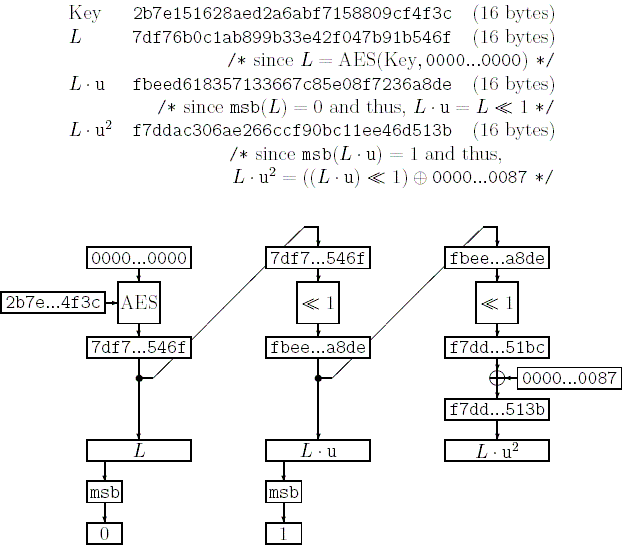The following picture gives the data flow in OMAC1, where AES with 128-bit key is used as the underlying blockcipher, and the message length is 40 bytes (Also available in [.ps] and [.pdf]).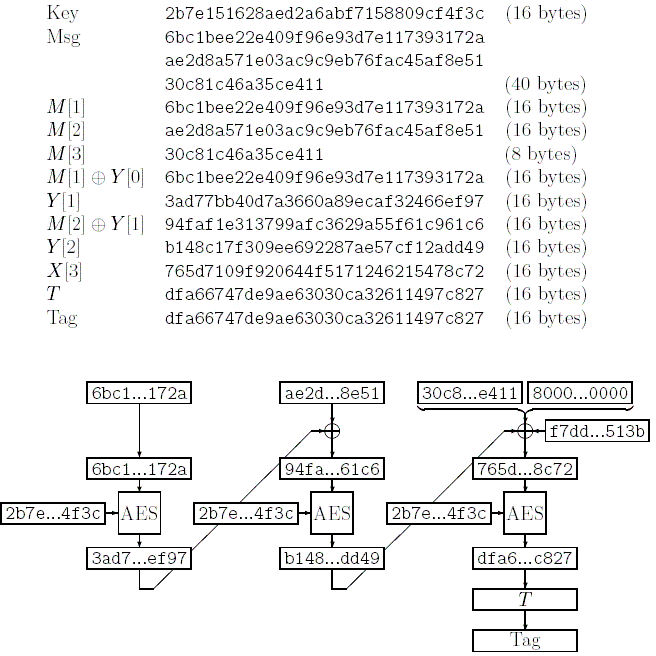OMAC: IP statement

There are no IP claims related to OMAC by me or, to the best of my knowledge, by anybody else. OMAC is entirely in the public domain.

Here is the IP statement sent to NIST (May 13, 2003) [.pdf].

 OMAC: Related works
• EMAC:
E. Petrank and C. Rackoff. CBC MAC for real-time data sources. J. Cryptology, vol. 13, no. 3, pp. 315--338, 2000.
• RMAC:
E. Jaulmes, A. Joux and F. Valette. On the security of randomized CBC-MAC beyond the birthday paradox limit: A new construction. Fast Software Encryption, FSE 2002, LNCS 2365, pp. 237--251, Springer-Verlag. Full version is posted on IACR ePrint server [www].
• XCBC:
J. Black and P. Rogaway. CBC MACs for arbitrary-length messages: The three key constructions. Advances in Cryptology --- CRYPTO 2000, LNCS 1880, pp. 197--215, Springer-Verlag.
• TMAC:
K. Kurosawa and T. Iwata. TMAC: Two-Key CBC MAC. Topics in Cryptology --- CT-RSA 2003, LNCS 2612, pp. 33--49, Springer-Verlag. Full version is posted on IACR ePrint server [www].
• EAX:
M. Bellare, P. Rogaway, and D. Wagner. A conventional authenticated-encryption mode. Fast Software Encryption, FSE 2004. Full version is posted on IACR ePrint server [www].

• NIST's modes of operation page is here [www].
• Phillip Rogaway maintains XCBC web page. His web page is here [www] and XCBC page is here [www].

 OMAC: Implementations
• Adder has kindly implemented OMAC. The website is here. The PHP source code is also available here.
• ``Secure Programming Cookbook for C and C++'' (from O'REILLY) written by John Viega and Matt Messier contains OMAC code. John Viega's web page is here [www] and O'REILLY's web page is here [www].
• Brian Gladman has published his OMAC code. His web page is here [www] and his implementation is here [www].
• Tom St Denis maintains his LibTomCrypt library. OMAC1 code is included. His implementation is here [www].
• Jack Lloyd maintains Botan. It is a C++ library implementing a variety of cryptographic algorithms and formats. OMAC is included. The implementation is available here [www].
• Paulo Barreto implemented EAX and OMAC in C++ and Java. The implementation is available here [www].
• Jose Luis Gomez Pardo added OMAC to the Mathematica implementation of AES. The implementation is available here [www].
• Wolfgang Ehrhardt implements various cryptographic primitives as an open source Pascal/Delphi code available in the AES zip library. The web site is here [www], and the implementation is available here [www].

To Iwata's web page.
Page created: May 1, 2006.
Last update: December 30, 2014.